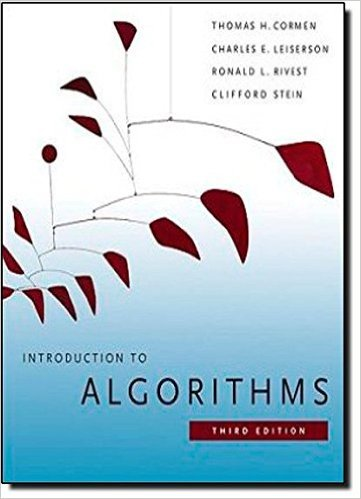×
Get Full Access to Introduction To Algorithms - 3 Edition - Chapter 16 - Problem 16-2
Get Full Access to Introduction To Algorithms - 3 Edition - Chapter 16 - Problem 16-2

×

# Scheduling to minimize average completion time Suppose youISBN: 9780262033848 130

## Solution for problem 16-2 Chapter 16

Introduction to Algorithms | 3rd Edition

• Textbook Solutions
• 2901 Step-by-step solutions solved by professors and subject experts
• Get 24/7 help from StudySoup virtual teaching assistantsIntroduction to Algorithms | 3rd Edition

4 5 1 287 Reviews
11
0
Problem 16-2

Step-by-Step Solution:
Step 1 of 3

Lesson 3.1 – Differential Rules F' (Derivative) c 0 x 1 xn n*x n­1 c*f(x) c*f '(x) f + g f ' + g' f ' ­ g' f ' ­ g' ex ex Examples 1) y = 3x + 2 2) y = x + 12x – 2x 4 3) y = e + 1000x y' = 3 + 0 y' = 12x +24x – 8x 3 y' = e + 1000 y' = 3 Lesson 3.2 – Product and Quotient Rule f ' (derivative) Product Rule: f * g f 'g + g'f Example: y = 4x*2x y' = (2x)*(4x)'+ (4x)*(2x)' y' = (4)*(2x) + (4x)*(2)

Step 2 of 3

Step 3 of 3